# Test: Class 10 Science: CBSE Sample Question Paper- Term I (2021-22)

## 60 Questions MCQ Test CBSE Sample Papers For Class 10 | Test: Class 10 Science: CBSE Sample Question Paper- Term I (2021-22)

Description
Attempt Test: Class 10 Science: CBSE Sample Question Paper- Term I (2021-22) | 60 questions in 40 minutes | Mock test for Class 10 preparation | Free important questions MCQ to study CBSE Sample Papers For Class 10 for Class 10 Exam | Download free PDF with solutions
QUESTION: 1

Solution:
QUESTION: 2

Solution:
QUESTION: 3

###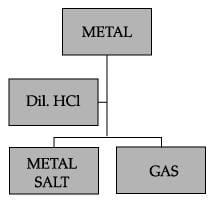Which of the following two combinations are correct?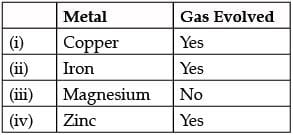Solution:
QUESTION: 4

Which of the following correctly represents a balanced chemical equation?

Solution:
QUESTION: 5

The graph given below depicts a neutralization reaction (acid + alkali → salt + water).
The pH of a solution changes as we add excess of acid to an alkali.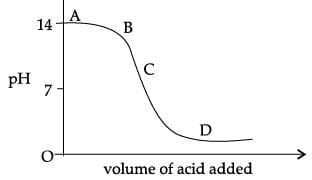Which letter denotes the area of the graph where both acid and salt are present?

Solution:
QUESTION: 6

In the reaction of iron with copper sulphate solution:
CuSO4 + Fe → Cu + FeSO4
Which option in the given table correctly represents the substance oxidised and the reducing agent?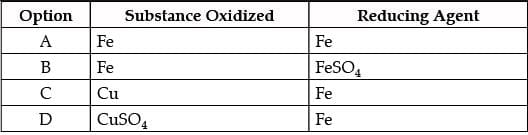Solution:
QUESTION: 7

The chemical reaction between copper and oxygen can be categorized as:

Solution:
QUESTION: 8

Which of the given options correctly represents the Parent acid and base of Calcium Carbonate?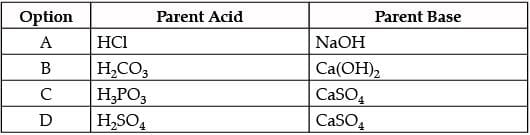Solution:
QUESTION: 9

How will you protect yourself from the heat generated while diluting a concentrated acid?

Solution:
QUESTION: 10

Why is it important to balance a skeletal chemical equation?

Solution:
QUESTION: 11

Carefully study the diagram of the human respiratory system with labels A, B, C and D. Select the option which gives correct identification and main function and /or characteristic.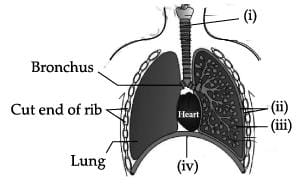Solution:
QUESTION: 12

Identify the option that indicates the correct enzyme that is secreted in location A, B and C.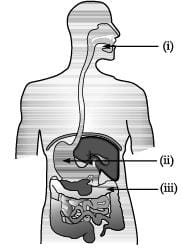Solution:
QUESTION: 13

Opening and closing of stomatal pore depends on:

Solution:
QUESTION: 14

The figure given below shows a schematic plan of blood circulation in humans with labels (i) to (iv). Identify the correct label with its functions?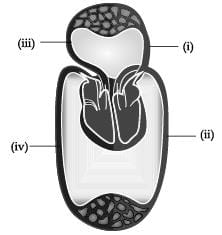Solution:
QUESTION: 15

Identify the phase of circulation which is represented in the diagram of heart given below. Arrows indicate contraction of the chambers shown.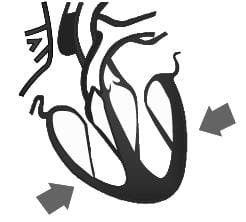Solution:
QUESTION: 16

Observe the diagram of Human digestive system.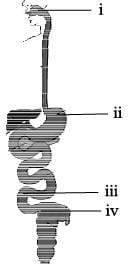Match the labeling referred in column I and correlate with the function in column II.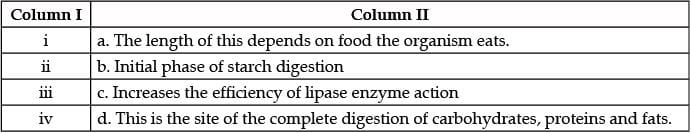Solution:
QUESTION: 17

Which of the following mirror is used by a dentist to examine a small cavity in a patient’s teeth?

Solution:
QUESTION: 18

Which diagram shows image formation of an object on a screen by a converging lens?

Solution: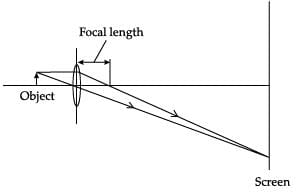QUESTION: 19

Which of the following can make a parallel beam of light when light from a point source is incident on it?

Solution:
QUESTION: 20

Consider these indices of refraction: glass: 1.52; air: 1.0003; water: 1.333. Based on the refractive indices of three materials, arrange the speed of light through them in decreasing order.

Solution:
QUESTION: 21

If a beam of red light and a beam of violet light are incident at the same angle on the inclined surface of a prism from air medium and produce angles of refraction r and v respectively, which of the following is correct?

Solution:
QUESTION: 22

Examine the above figure and state which of the following option is correct? [one small box in the figure is equal to 1 cm]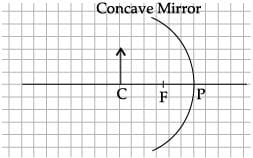Solution:
QUESTION: 23

The angle of incidence from air to glass at the point O on the hemispherical glass slab is.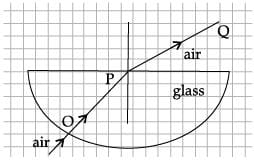Solution:
QUESTION: 24

A prism ABC (with BC as base) is placed in different orientations. A narrow beam of white light is incident on the prism as shown in below Figure. In which of the following diagrams, after dispersion, the third colour from the top of the spectrum corresponds to the colour of the sky?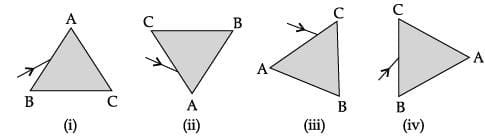Solution:
QUESTION: 25

Shyam heated a small amount of light green colored compound X in a test tube.  He found that the compound losed some water and then gas Z with suffocating smell comes out. The vapors of gas are collected and dissolved in water. The solution turns blue litmus red. The residue Y left in the test tube turns reddish brown.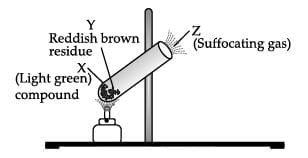Identify X,Y and Z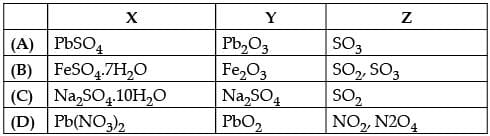Solution:

The green colour compound X is FeSO4.7H2O.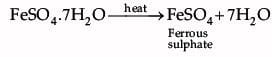Ferrous sulphate decomposes with the evolution of a gas having a typical odour of burning sulphur. It is a thermal decomposition reaction.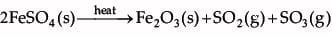SO2 and  SO3  are acidic in nature, turn blue litmus red as they react with water to form acids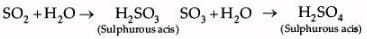Hence, X is FeSO4.7H2O , Y is Fe2O3 and Z is SO2, SO4

QUESTION: 26

Choose the correct option .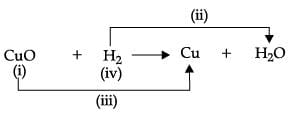Solution:

In the given equation, label (i) represent oxidizing agent, (ii) is oxidation, (iii) is reduction and (iv) represents reducing agent.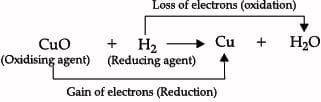QUESTION: 27

Study the given experimental set-up carefully. Based on the diagram, Choose the correct observation(s) ?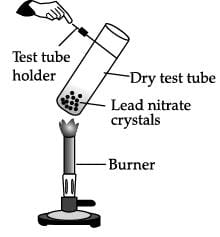Solution:

On heating, lead nitrate decomposes to give yellow lead monoxide, nitrogen dioxide (emission of brown fumes) and oxygen gas.  The reaction that takes place is :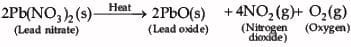QUESTION: 28

The given experimental set up is used to test a few solutions which contain hydrogen but are not categorized as acids.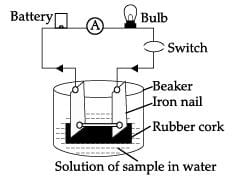Which of these observation is incorrect?

Solution:

Glucose and ethanol do not conduct electricity while NaOH and HCl conduct electricity as they produce ions in the solution.

QUESTION: 29

An electrolytic cell consists of
(i) positively charged cathode
(ii) negatively charged anode
(iii) positively charged anode
(iv) negatively charged cathode

Solution:

Positively charged ions are called cations as they are deposited at negatively charged pole. Negatively charged ions are called anions as these are deposited at positively charged pole. That’s why the negatively charged pole is called cathode and positively charged pole is called anode.

QUESTION: 30

An aqueous solution turns red litmus solution blue. Excess addition of which of the following solution would reverse the change?

Solution:

The solution turns red litmus to blue it means that it is basic in nature, to reverse the change acid should be added to it. Thus, out of the given options hydrochloric acid is the correct answer.

QUESTION: 31

Directions: Assertion (A) and Reason (R). Answer these questions selecting the appropriate option given below:
Assertion (A): An equation is the shorthand representation of a chemical reaction.
Reason (R): A chemical reaction is a process in which a chemical substance is transformed into another chemical substance.

Solution:

A chemical reaction is a process in which a chemical substance is transformed into another chemical substance. A chemical equation is the symbolic representation of a chemical reaction in the form of symbols and formulae. It is a way to represent the chemical reaction in a concise and informative way.

QUESTION: 32

Directions: Assertion (A) and Reason (R). Answer these questions selecting the appropriate option given below:
Assertion (A): Sodium carbonate is a basic salt.
Reason (R): It is a salt of weak acid and strong base.

Solution:

Sodium carbonate is a basic salt because it is a salt of weak acid and strong base.

QUESTION: 33

Directions: Assertion (A) and Reason (R). Answer these questions selecting the appropriate option given below:
Assertion (A): The by product of autotrophic nutrition is oxygen.
Reason (R): Oxygen is released into the atmosphere through stomata.

Solution:

Plants require sunlight, water, chlorophyll and carbon dioxide as raw materials for photosynthesis. Oxygen is released as by-product through stomata on leaf.

QUESTION: 34

Directions: Assertion (A) and Reason (R). Answer these questions selecting the appropriate option given below:
Assertion (A): A beam of white light gives a spectrum on passing through a glass prism.
Reason (R): Speed of light outside the prism is different from the speed of light inside the prism.

Solution:

A beam of white light gives a spectrum on passing through a glass prism. The band of seven colours formed due to dispersion of white light is called spectrum. Dispersion takes place because the refractive index of medium for different colour is different. Therefore when white light travels from air to air, refractive index remains same and no dispersion occurs.

QUESTION: 35

The gas produced when dil. HCl is added to a reactive metal:

Solution:

Hydrogen gas is produced when dilute HCl is added to a reactive metal.

QUESTION: 36

On the basis of sequence of reactions, identify the most and least reactive elements.
A + BX → AX + B
C + AY → CY + A

Solution:

The most reactive metal is C and the least reactive metal is B.

QUESTION: 37

Choose the correct statement.

Solution:

ATP is the higher energy form (the recharged battery) while ADP is the lower energy form. Respiration is catabolic while photosynthesis is an anabolic process. In respiration, glucose is broken down into water and carbon dioxide (and energy). In contrast carbon dioxide and water combine in the presence of sunlight to produce glucose and oxygen during photosynthesis.

QUESTION: 38

Which of the following chemical reactions is incorrect?

Solution:

Pyruvate is converted into lactic acid when there is a lack of oxygen in our muscle cells.

QUESTION: 39

Which of the following take place after we exercise?

Solution:

Our body needs more energy when we do exercise. We get energy by the oxidation of food. Due to exercise the body is able to get rid of excess carbon dioxide.

QUESTION: 40

Study the given experimental set up.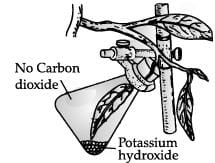The aim of the given experiment is to prove that _________ is essential for the process of photosynthesis.

Solution:

The experimental set up is used to prove that carbon dioxide is essential for photosynthesis.

QUESTION: 41

The gas X in the given graph is _______.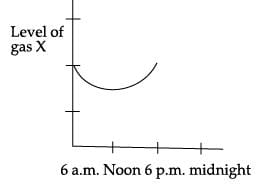Solution:

Green plants prepare food by taking CO2 from the air by the process of photosynthesis. Photosynthesis occurs more at noon. Hence the carbon dioxide gas is less during noon.

QUESTION: 42

In case of concave mirror, the image  distance is _________ when image is formed in front of the mirror and   _________________  when the image is formed behind the mirror.

Solution:

If an image formed behind the concave mirror, the object distance is positive but if an image is formed in front of the mirror, the image distance is negative.

QUESTION: 43

A negative sign in the magnification value indicate that the image is _________

Solution:

A negative sign in the magnification value indicate that the image is real and inverted.

QUESTION: 44

An image formed by concave mirror is virtual, when the object is placed:

Solution:

An image formed by concave mirror is virtual, when the object is placed between P and F.

QUESTION: 45

In which of the following, the image of an object placed at infinity will be highly diminished and point sized?

Solution:

All the incident rays will be parallel to the principal axis and after reflection or refraction they actually meet or appear to meet at the principal focus.

QUESTION: 46

A student obtained a sharp image of a candle flame placed at the distant end of the laboratory table on a screen using a concave mirror to determine its focal length. The teacher suggested him to focus a distant building about 1 km far from the laboratory, for getting more correct value of the focal length. In order to focus the distant building on the same screen the student should slightly move the:

Solution:

The object is at infinity , so to obtain sharp image screen should be moved towards mirror.

QUESTION: 47

In your laboratory you trace the path of light rays through a glass slab for different values of angle of incidence (∠i) and in each case measure the values of the corresponding angle of refraction (∠r) and angle of emergence (∠e).

Solution:

When a ray of light passes through the glass slab, then the angle of incidence is found to be nearly equal to angle of emergence and greater than angle of refraction.

QUESTION: 48

You are given water, mustard oil, glycerine, and kerosene. In which of these media, a ray of light incident obliquely at some angle would bend the most.

Solution:

Out of the given four materials, the refractive index of glycerine is highest. So, greatest deviation of incident light ray is observed in case of glycerine.

QUESTION: 49

CASE-I
Chemistry in Automobiles:
For an internal combustion engine to move a vehicle down the road, it must convert the energy stored in the fuel into mechanical energy to drive the wheels. In your car,the distributor and battery provide this starting energy by creating an electrical "spark",which helps in combustion of fuels like gasoline. Below is the reaction depicting complete combustion of gasoline in full supply of air:
2C8H18(l) + 25O2(g) → 16 'X' + Y
Which of the following are the products obtained from the reaction mentioned in the above case?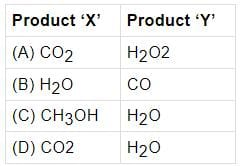Solution:

The complete combustion of gasoline in full supply of air results in production of carbon dioxide and water. The chemical reaction is as follows:
2C8H18 (l) + 25O2(g) → 16CO2(g) + 18H2O(g)

QUESTION: 50

CASE-I
Chemistry in Automobiles:
For an internal combustion engine to move a vehicle down the road, it must convert the energy stored in the fuel into mechanical energy to drive the wheels. In your car,the distributor and battery provide this starting energy by creating an electrical "spark",which helps in combustion of fuels like gasoline. Below is the reaction depicting complete combustion of gasoline in full supply of air:
2C8H18(l) + 25O2(g) → 16 'X' + Y
Identify the types of chemical reaction occurring during the combustion of fuel:

Solution:

The addition of oxygen to a substance or removal of hydrogen from a substance is called oxidation. The reaction in which the heat energy is produced is called exothermic reaction.

QUESTION: 51

CASE-I
Chemistry in Automobiles:
For an internal combustion engine to move a vehicle down the road, it must convert the energy stored in the fuel into mechanical energy to drive the wheels. In your car,the distributor and battery provide this starting energy by creating an electrical "spark",which helps in combustion of fuels like gasoline. Below is the reaction depicting complete combustion of gasoline in full supply of air:
2C8H18(l) + 25O2(g) → 16 'X' + Y
On the basis of evolution/absorption of energy, which of the following processes are similar to combustion of fuel?
(i) Photosynthesis in plants
(ii) Respiration in the human body
(iii) Decomposition of vegetable matter
(iv) Decomposition of ferrous sulphate.

Solution:

The process of respiration in the human body and decomposition of vegetable matter involves evolution of energy.

QUESTION: 52

CASE-I
Chemistry in Automobiles:
For an internal combustion engine to move a vehicle down the road, it must convert the energy stored in the fuel into mechanical energy to drive the wheels. In your car,the distributor and battery provide this starting energy by creating an electrical "spark",which helps in combustion of fuels like gasoline. Below is the reaction depicting complete combustion of gasoline in full supply of air:
2C8H18(l) + 25O2(g) → 16 'X' + Y
‘A student while walking on the road observed that a cloud of black smoke belched out from the exhaust stack of moving trucks on the road.’ Choose the correct reason for the production of black smoke:

Solution:

The limited supply of air leads to incomplete combustion of fuel, which in turn leads to the production of black smoke.

QUESTION: 53

CASE-II
Study the diagram of human respiratory system and answer the following questions.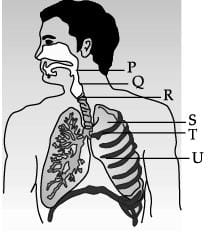The balloon like structures present in ‘S’ is:

Solution:

The balloon like structure are alveoli. Alveoli are air sacs at the end of bronchioles. They allow oxygen and carbon dioxide to move between the lungs and the blood-stream.

QUESTION: 54

CASE-II
Study the diagram of human respiratory system and answer the following questions.Which of these organ is surrounded by cartilaginous rings?

Solution:

R (Trachea) is supported by rings of cartilage.

QUESTION: 55

CASE-II
Study the diagram of human respiratory system and answer the following questions.Which of these statements is incorrect regarding human lungs?

Solution:

Lungs are the primary breathing organ. It is the main respiratory surface available for the exchange of gases (O2 & CO2).

QUESTION: 56

CASE-II
Study the diagram of human respiratory system and answer the following questions.Which of these is the function of balloon like structure present in lungs?

Solution:

The balloon like structure called alveoli allow oxygen and carbon dioxide to move between the lungs and the blood-stream.

QUESTION: 57

CASE-III
A beam of white light falling on a glass prism gets split into seven colours marked 1 to 7. Study the diagram and answer the following questions.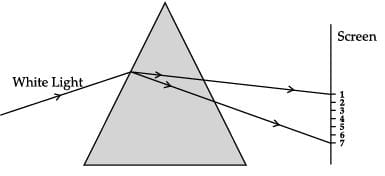The colour at positions marked 1 and 3 are similar to the colour of ‘turmeric’ and the colour of ‘Chilli powder’, respectively. Choose the correct option.

Solution:

The colour at position marked 1 is red while colour at position marked 3 is yellow in colour.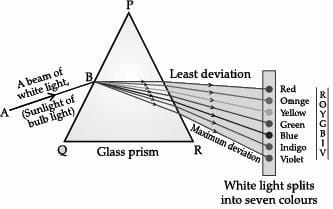QUESTION: 58

CASE-III
A beam of white light falling on a glass prism gets split into seven colours marked 1 to 7. Study the diagram and answer the following questions.Which two positions correspond to the colour of solution of copper sulphate and signal used to move the vehicles?

Solution:

Copper sulphate is blue in colour, which is at position 5 where the signal used to move the vehicle is green. Green is at position 5.

QUESTION: 59

CASE-III
A beam of white light falling on a glass prism gets split into seven colours marked 1 to 7. Study the diagram and answer the following questions.I. Light of colour of chilli powder bends the most.
II. The light of colour of brinjal bends the least.
Which of these statement(s) is incorrect?

Solution:

Both the statements are incorrect. Violet bends the most.

QUESTION: 60

CASE-III
A beam of white light falling on a glass prism gets split into seven colours marked 1 to 7. Study the diagram and answer the following questions.Which of these marked colour deviates the least after refraction through a prism ?

Solution:

When a beam of white light gets refracted through a glass prism, it refracts through different angles causing a splitting of white light into its seven constituent colours (VIBGYOR). This gives rise to the formation of the colour spectrum. Violet colour (marked as 7) deviate the most and red colour (marked as 1) deviates the least after refraction through a prism.Use Code STAYHOME200 and get INR 200 additional OFF Use Coupon Code